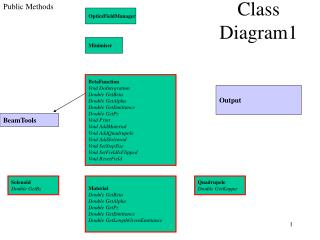DownloadDownload PresentationClass Diagram 1

Class Diagram 1

Télécharger la présentationClass Diagram 1

- - - - - - - - - - - - - - - - - - - - - - - - - - - E N D - - - - - - - - - - - - - - - - - - - - - - - - - - -
Presentation Transcript

1. Public Methods OpticsFieldManager Class Diagram1 Minimiser BetaFunction Void DoIntegration Double GetBeta Double GetAlpha Double GetEmittance Double GetPz Void Print Void AddMaterial Void AddQuadrupole Void AddSolenoid Void SetStepSize Void SetFieldIsFlipped Void ResetField Output BeamTools Solenoid Double GetBz Material Double GetBeta Double GetAlpha Double GetPz Double GetEmittance Double GetLengthGivenEmittance Quadrupole Double GetKappa

2. Solenoid • Define • Builds a vector of BTSheet • Would use BTSolenoid but it needs rewriting • OpticsSolenoid calculates on-axis Bz only • BTSolenoid insists on calculating a full field map (slow!!) • I have a quicker algorithm anyway which I may use • Returns it to BetaFunction • This should be better integrated with BeamTools

3. Quadrupole • Define length, z-position (maybe aperture later) • Get kappa w/o fringe fields, i.e. square edge approx • Kappa is focusing strength • Returns kappa or -kappa depending on whether I am looking at x axis or y axis • Should probably include fringe fields at some stage • A neater algorithm would be to calculate dB/dr on-axis from a field map • Slower, maybe appropriate for second approximation to linear problem

4. Material • Define material, length, position • Gives changes in optical functions on passing through a material • Uses linear approximation (fairly good) • This is something Bravar hasn’t done • Already beating them • Formulae come from • CERN/PS 2001-0013 (AE) DESCRIPTION OF BEAM-MATTER INTERACTION IN THE COVARIANCE MATRIX FORMALISM • Beware of binomial approximation in some results! • Works in both directions, forward and backward

5. BetaFunction • Builds an optical geometry • AddOpticalComponent type methods • Use a field map • Calculates focusing strength kappa from quadrupole and on-axis Bz • Calculates optical functions • Then run DoIntegration() to calculate beta • Beta etc stored as array at intervals dZ (dZ +ve or -ve) • Use GetOpticalFunction() to get linear interpolation for the optical functions, pz etc • Maybe use BTSpline at some stage

6. Minimiser • Under Construction! • Need to learn how Minuit works • Initial thoughts/aims • Constraints are beta and alpha at various points down the beamline • Variable parameters are optical parameters, e.g. current in solenoids • Other possibilities include material thickness, quad dB/dr, etc • Score according to?? • Then some sort of manager class/executable?